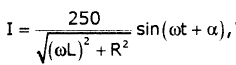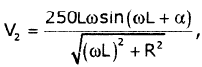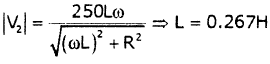Courses

# Networks - MCQ Test - 1

## 15 Questions MCQ Test GATE Electrical Engineering (EE) 2022 Mock Test Series | Networks - MCQ Test - 1

Description
This mock test of Networks - MCQ Test - 1 for GATE helps you for every GATE entrance exam. This contains 15 Multiple Choice Questions for GATE Networks - MCQ Test - 1 (mcq) to study with solutions a complete question bank. The solved questions answers in this Networks - MCQ Test - 1 quiz give you a good mix of easy questions and tough questions. GATE students definitely take this Networks - MCQ Test - 1 exercise for a better result in the exam. You can find other Networks - MCQ Test - 1 extra questions, long questions & short questions for GATE on EduRev as well by searching above.
QUESTION: 1

###If each branch of a 'Y' circuit has impedance equal tom , then each branch of the equivalent delta circuit has impedance equal to

Solution: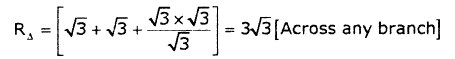QUESTION: 2

### The transmission parameters for circuit shown below are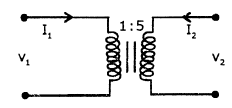Solution: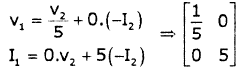QUESTION: 3

### The rise time of the RC circuit shown below is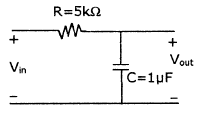Solution:
QUESTION: 4

A station in a network forwards incoming packets by placing them on its shortest output queue. What routing algorithm is being used?

Solution:
QUESTION: 5

A tree of the graph shown below is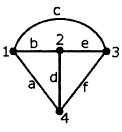Solution: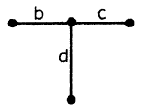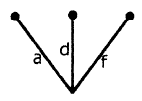QUESTION: 6

The driving point function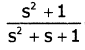can be realized as a

Solution:
QUESTION: 7

The equivalent resistance between A and B in the following network is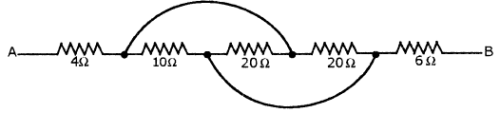Solution:

The equivalent network is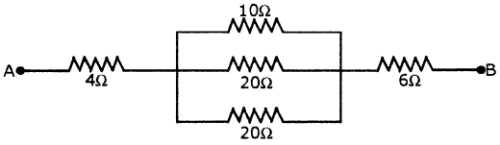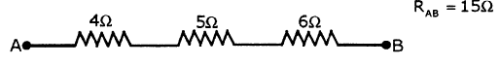QUESTION: 8

The network shown below is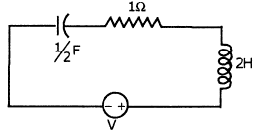Solution:
1. Roots are complex conjugates
QUESTION: 9

The impendance matrix for the circuit shown below is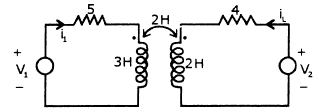Solution:
QUESTION: 10

The steady state voltage across the capacitors C1, C2 and C3 shown in the circuit below are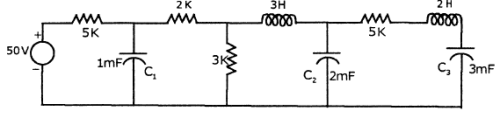Solution:

Voltage across C1 under steady state =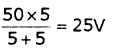Voltage across C2 is voltage across 31d/ resistance =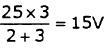voltage across C3 is equal to voltage across C2 under steady state = 15V

QUESTION: 11

Which of the following is correct?

1. Cut set is the number of branches that are to be removed to break the graph into two parts.

2. Rank of cut set matrix = Rank of incidence matrix

Adding one twig to the existing co-tree results in one tie-set.

Solution:

Cut set is minimum number of branches to be removed to break graph into two parts.
Adding a link to existing tree results in one positive set

QUESTION: 12

Find the voltage Vx in the given[/expand] circuit.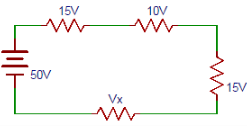Solution:

From the circuit applying Kirchhoff’s voltage law, we can write 50= 15+ 10+ 15+Vx => Vx= 10V.

QUESTION: 13

The Dual of the network shown below is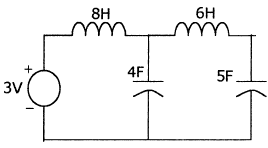Solution:
QUESTION: 14

Calculate the power dissipated in 3.Ω resistor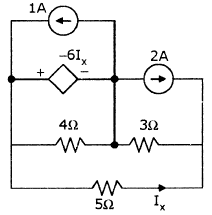Solution:
QUESTION: 15

In the circuit shown below, Vs = 250 sin 500t,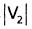= 200. If R = 100Ω, then the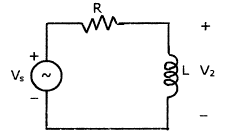Solution: